# 结构体

## 1、结构体定义和使用

#include <iostream>
#include "swap.h"
using namespace std;
#define week 7
#include <string>

struct student
{
//名字
string name;
//年龄
int age;
//分数
int score;
};

int main()
{
//第一种
struct student s1;
s1.name = "张同学";
s1.age = 34;
s1.score = 80;
cout << "姓名：" << s1.name << "" << s1.age << "" << s1.score << endl;
//第二种
struct student s2 = {"二涛",32,85};
cout << "姓名：" << s2.name << "" << s2.age << "" << s2.score << endl;

system("pause");
return 0;
}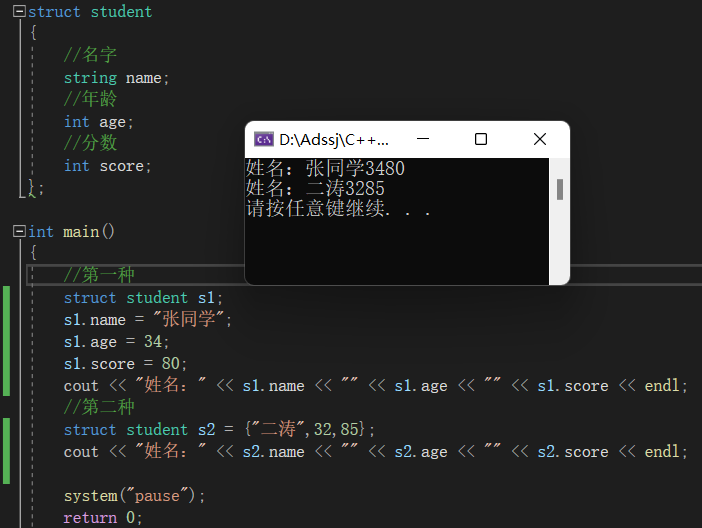## 2、结构体数组

#include <iostream>
#include "swap.h"
using namespace std;
#define week 7
#include <string>

struct student
{
//名字
string name;
//年龄
int age;
//分数
int score;
};

int main()
{

struct student stuary =
{
{"小明",20,80},
{"小亮",20,85},
{"小华",20,89},
};
for (int i = 0; i < 3; i++)
{
cout << "name = " << stuary[i].name << endl;
cout << "age =" << stuary[i].age << endl;
cout << "score =" << stuary[i].score << endl;
}

system("pause");
return 0;
}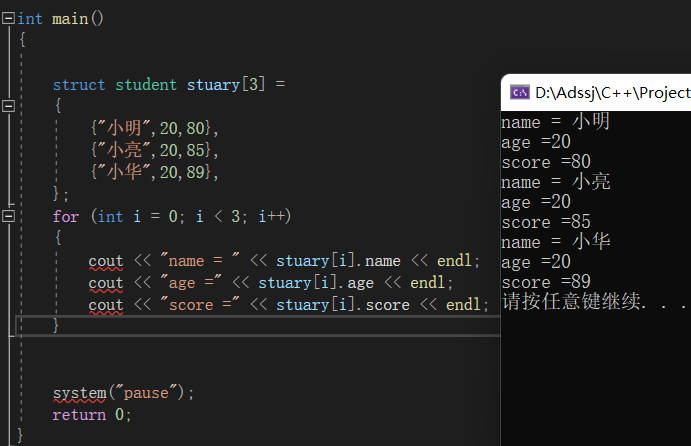## 3、结构体指针

#include <iostream>
#include "swap.h"
using namespace std;
#define week 7
#include <string>

struct student
{
//名字
string name;
//年龄
int age;
//分数
int score;
};

int main()
{

struct student stuary =
{
{"小明",20,80},
{"小亮",20,85},
{"小华",20,89},
};

struct student* p = &stuary;
cout << "name=" << p->name << "age=" << p->age << "score=" << p->score << endl;

system("pause");
return 0;
}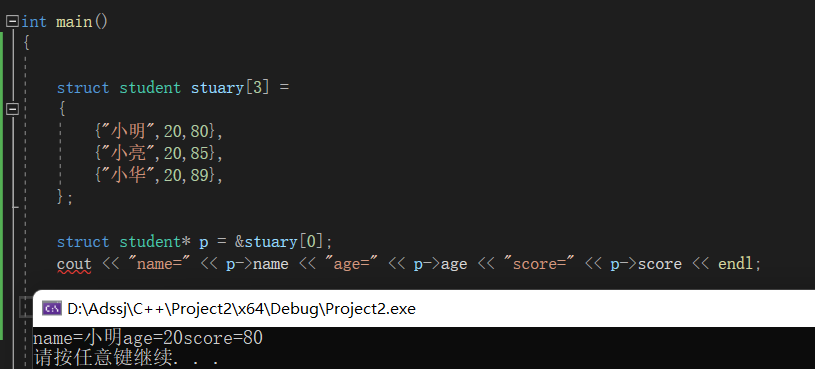## 4、结构体做函数参数

#include <iostream>
#include "swap.h"
using namespace std;
#define week 7
#include <string>
struct student
{
//名字
string name;
//年龄
int age;
//分数
int score;
};
void print_sty(struct student s)
{
cout << "值传递 s.name=" << s.name << "s.age=" << s.age << "s.score=" << s.score << endl;
}

void print_sty_2(struct student *p)
{
cout << "地址传递s.name=" << p->name << "s.age=" << p->age << "s.score=" << p->score << endl;
}
int main()
{
struct student stuary =
{
{"小明",20,80},
{"小亮",20,85},
{"小华",20,89},
};

// 1、值传递
print_sty(stuary);
// 2、地址传递
struct student *p = &stuary;
print_sty_2(p);
system("pause");
return 0;
}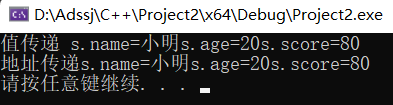## 5、结构体嵌套结构体

#include <iostream>
#include "swap.h"
using namespace std;
#define week 7
#include <string>

struct student
{
//名字
string name;
//年龄
int age;
//分数
int score;
};
struct teacher
{
int id;
string name;
int age;
struct student student1;
};

int main()
{

struct student stuary =
{
{"小明",20,80},
{"小亮",20,85},
{"小华",20,89},
};

struct student* p = &stuary;
//cout << "name=" << p->name << "age=" << p->age << "score=" << p->score << endl;

teacher t = { 100,"老徐",50,stuary };
cout << "id=" << t.id << "name=" << t.name << "age=" << t.age
<< "t.student1.name=" << t.student1.name << "t.student1.age=" << t.student1.age << "t.student1.score=" << t.student1.score <<endl;
system("pause");
return 0;
}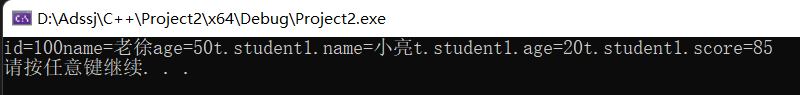## 6、结构体中const使用场景

#include <iostream>
#include "swap.h"
using namespace std;
#define week 7
#include <string>
struct student
{
//名字
string name;
//年龄
int age;
//分数
int score;
};
void printstu(const student *p)
{
// 加 const 禁止修改，防止误操作
cout << "name=" << p->name << "age=" << p->age << "score=" << p->score << endl;
}
int main()
{
struct student stuary =
{
{"小明",20,80},
{"小亮",20,85},
{"小华",20,89},
};
printstu(&stuary);
system("pause");
return 0;
}



# 结构体案例

#include <iostream>
#include "swap.h"
using namespace std;
#define week 7
#include <string>

struct student
{
//名字
string name;
//分数
int score;
};
struct teacher
{
string name;
struct student stu;
};

void allinfo(struct teacher t[], int len)
{
string nameseed = "ABCDEF";
for (int i = 0; i < len; i++)
{
t[i].name = "teacher";
t[i].name += nameseed[i];
for (int j = 0; j < 5; j++)
{
t[i].stu[j].name = "stdent_";
t[i].stu[j].name += nameseed[i];
int random = rand() % 60+40;
t[i].stu[j].score = random;
}
}
}
void printinfo(struct teacher t[], int len)
{
for (int i = 0; i < len; i++)
{
cout << "name=" << t[i].name;
for (int j = 0; j < 5; j++)
{
cout << "学生name=" << t[i].stu[j].name;
cout << "学生score=" << t[i].stu[j].score << endl;
}
}
}
int main()
{
struct teacher teacherary;
allinfo(teacherary, 3);
printinfo(teacherary, 3);

system("pause");
return 0;
}


07-02
03-22
06-14
07-16
05-21
10-04
08-12
05-06
05-26519

### “相关推荐”对你有帮助么？

•非常没帮助
•没帮助
•一般
•有帮助
•非常有帮助被折叠的  条评论 为什么被折叠?到【灌水乐园】发言27878678678

¥2 ¥4 ¥6 ¥10 ¥20余额支付 (余额：-- )扫码支付获取中扫码支付点击重新获取扫码支付1.余额是钱包充值的虚拟货币，按照1:1的比例进行支付金额的抵扣。
2.余额无法直接购买下载，可以购买VIP、C币套餐、付费专栏及课程。余额充值